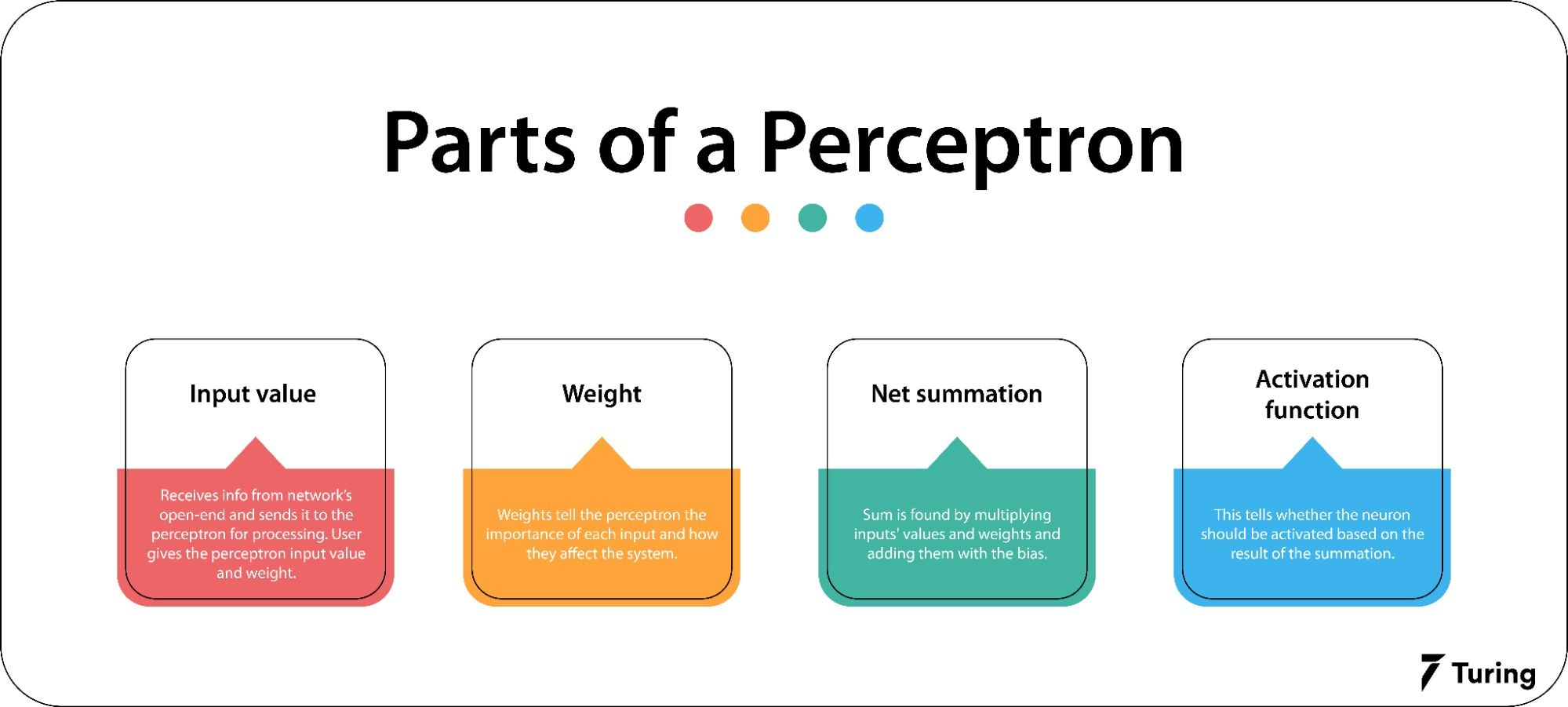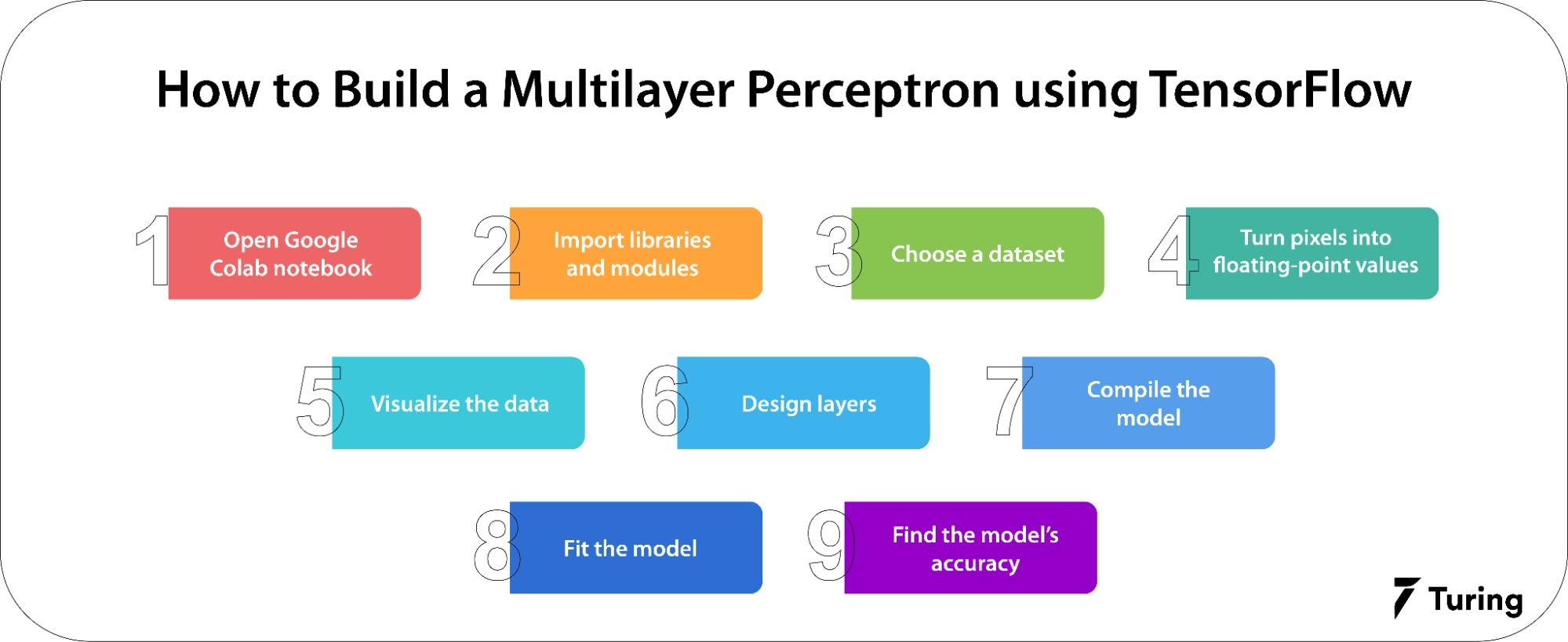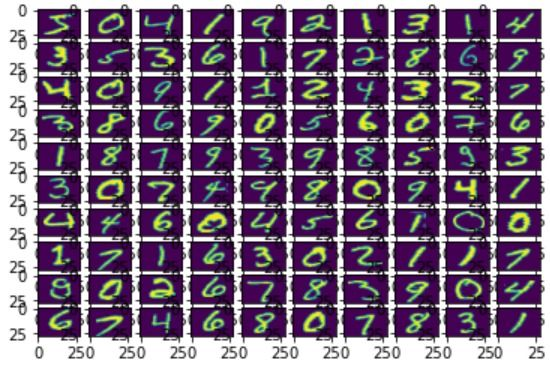# How to Make a Simplified Multilayer Perceptron in TensorFlowBuilding a neural network takes time and skill, but frameworks can make things much simpler. In fact, using frameworks in ML projects is easy when you build a multilayer perceptron (MLP) in TensorFlow. This article will discuss neural networks, MLPs, and how to build one in TensorFlow using Google Colab.

## What is a multilayer perceptron?

A multilayer perceptron in deep learning is the most complicated neural network architecture. It is made up of many layers of perceptrons. These perceptrons are algorithms that mimic how the brain can recognize and tell the difference between a series of events. MLPs are the building blocks of neural networks, but they are usually made to see how a computer learns.

Frank Rosenbluth proposed the first type of machine learning model back in 1958. It was called a single-layer perceptron or SLP. It is the simplest part of a neural network and is used to spread learning. It only looks at a linear pattern by itself. Although simple, an SLP has small parts similar to how an atom is made up of smaller parts.

On the other hand, a multilayer perceptron is made by connecting several single-layer perceptrons and studying their relationships simultaneously. Note that the number of SLPs connected to a neural network influences its complexity.

## How do perceptrons function?

Perceptrons are made when you instruct a machine to do something. Each input is given a weight based on how important it is to the network. The total weight of all the inputs affects the machine's behavior.

The learning model covers everything from what the machine is given to how it acts in the end. This final behavior is the perceptron.A perceptron has four major parts: input value or input layer, weight, net summation, and activation function.

### Input value

The input layer gets the information from the network’s open-end and sends it to the perceptron to be processed. The user gives the perception the input value and weight.

### Weight

Inputs are given weights. This tells the perceptron how important each input is and how much each input affects the whole system.

### Net summation

A simple perceptron usually receives multiple inputs. The sum is found by multiplying the inputs by the weights and then adding the sum with the bias. This sum is used to start the function that does something.

### Activation function

This part of the perceptron tells the neuron whether it should be activated. It looks at the result of the summation to decide what the neuron output will be.

## Implementing multilayer perceptron algorithmFollowing are the steps to implement a multilayer perceptron model.

### Step 1: Open Google Colab notebook

Select a new notebook and name it.

### Step 2: Import libraries and modules

The commands below will import the required library into the Google Colab environment.

``````import tensorflow as t
import numpy as np
from tensorflow.keras.models import Sequential
from tensorflow.keras.layers import Flatten
from tensorflow.keras.layers import Dense
from tensorflow.keras.layers import Activation
import matplotlib.pyplot as pt

``````

Here, we'll use the MNIST dataset to show how it works. It can be used as a train and test dataset immediately as it is built into TensorFlow.

``````(x_train, y_train), (x_test, y_test) = t.keras.datasets.mnist.load_data()

``````

Output:

``````11493376/11490434 [==============================] - 0s 0us/step
11501568/11490434 [==============================] - 0s 0us/step

``````

### Step 4: Turn pixels into floating-point values

In this step, we will turn the pixel values into floating-point values to make the predictions. Changing the numbers to grayscale values will help because they will get smaller and make the math easier and faster. As the values of pixels range from 0 to 256, everything except 0 is 255. If we divide all the numbers by 255, it will go from 0 to 1.

``````x_train = x_train.astype('float32')
x_test = x_test.astype('float32')

# For normalization image pixel values are divided by 255
gray_scale = 255
x_train /= gray_scale
x_test /= gray_scale

# To understand the structer of dataset
print("Feature matrix:", x_train.shape)
print("Target matrix:", x_test.shape)
print("Feature matrix:", y_train.shape)
print("Target matrix:", y_test.shape)
Outut:
Feature matrix: (60000, 28, 28)
Target matrix: (10000, 28, 28)
Feature matrix: (60000,)
Target matrix: (10000,)

``````

We know that the training dataset has 60,000 records, the test dataset has 10,000 records, and every image in the dataset is 2828.

### Step 5: Visualize the data

Code:

``````fig, ax = pt.subplots(10, 10)
k = 0
for i in range(10):
for j in range(10):
ax[i][j].imshow(x_train[k].reshape(28, 28),
aspect='auto')
k += 1
pt.show()

``````

Output:### Step 6: Make input, hidden, and output layers

The following points should be kept in mind when designing the layers:

• The sequential model lets us build models layer by layer as needed in a multilayer perceptron, but it only works for stacks of layers with one input and one output.
• ‘Flatten’ flattens the input without changing the size of the batch. For example, if the shape of the inputs is (batch size) but there is no feature axis, flattening adds a new channel dimension, and the shape of the output will be (batch size, 1).
• The sigmoid activation function is used in the activation step.
• The first two dense layers are hidden and are used to make a fully connected model.
• The output layer - the last dense layer - has 10 neurons that decide which category the image belongs to.

Code:

``````model = Sequential([

# reshape 28 row * 28 column data to 28*28 rows
Flatten(input_shape=(28, 28)),

# dense layer 1
Dense(256, activation='sigmoid'),

# dense layer 2
Dense(128, activation='sigmoid'),

# output layer
Dense(10, activation='sigmoid'),
])

``````

### Step 7: Compile the model

The compile function is employed here. It involves the use of loss, optimizers, and metrics. Sparse_categorical_crossentropy is used as the loss function and adam is used as the optimizer.

``````model.compile(optimizer='adam',
loss='sparse_categorical_crossentropy',
metrics=['accuracy'])

``````

### Step 8: Fit the model

Some important points to note in this step are:

• Epochs tell how many forward and backward passes the model will go through.
• Batch size is the number of samples. If the batch size is not set, 32 will be used by default.
• The value of split is a float between 0 and 1. At the end of each epoch, the model will set aside this amount of the training data to look at the loss and any other model metrics. This data won't be used to train the model.
``````model.fit(x_train, y_train, epochs=10,
batch_size=2000,
validation_split=0.2)

``````

Output:

``````Epoch 1/10
24/24 [==============================] - 4s 114ms/step - loss: 2.0474 - accuracy: 0.4557 - val_loss: 1.6607 - val_accuracy: 0.7004
Epoch 2/10
24/24 [==============================] - 1s 54ms/step - loss: 1.3223 - accuracy: 0.7373 - val_loss: 0.9816 - val_accuracy: 0.8207
Epoch 3/10
24/24 [==============================] - 1s 51ms/step - loss: 0.8212 - accuracy: 0.8249 - val_loss: 0.6347 - val_accuracy: 0.8712
Epoch 4/10
24/24 [==============================] - 1s 51ms/step - loss: 0.5745 - accuracy: 0.8689 - val_loss: 0.4709 - val_accuracy: 0.8913
Epoch 5/10
24/24 [==============================] - 1s 50ms/step - loss: 0.4527 - accuracy: 0.8882 - val_loss: 0.3890 - val_accuracy: 0.9022
Epoch 6/10
24/24 [==============================] - 1s 50ms/step - loss: 0.3861 - accuracy: 0.8999 - val_loss: 0.3429 - val_accuracy: 0.9080
Epoch 7/10
24/24 [==============================] - 1s 51ms/step - loss: 0.3449 - accuracy: 0.9073 - val_loss: 0.3121 - val_accuracy: 0.9154
Epoch 8/10
24/24 [==============================] - 2s 80ms/step - loss: 0.3165 - accuracy: 0.9128 - val_loss: 0.2901 - val_accuracy: 0.9208
Epoch 9/10
24/24 [==============================] - 2s 77ms/step - loss: 0.2947 - accuracy: 0.9180 - val_loss: 0.2731 - val_accuracy: 0.9243
Epoch 10/10
24/24 [==============================] - 1s 51ms/step - loss: 0.2772 - accuracy: 0.9222 - val_loss: 0.2587 - val_accuracy: 0.9278
<keras.callbacks.History at 0x7fc3f9dc7250>

``````

### Step 9: Find the accuracy of the model

The code to find the model accuracy is:

``````results = model.evaluate(x_test, y_test, verbose = 0)
print('test loss, test acc:', results)

``````

Code source

Output:

``````test loss, test acc: [0.2645658850669861, 0.9247000217437744]

``````

We used the model to make sure that it was accurate 92 percent of the time by evaluating the sample test data.

In this multilayer perceptron tutorial, we explored how an MLP functions and how to build one. The article provides a good start for individuals who want to know how to implement MLP using TensorFlow. This is especially true for developers and machine learning experts as perceptrons and TensorFlow play an important role in ML projects.

## Author

•### Turing

Author is a seasoned writer with a reputation for crafting highly engaging, well-researched, and useful content that is widely read by many of today's skilled programmers and developers.

The single-layer perceptron is the simplest neural network. It does not contain any hidden layer. It is used to look at how a machine learns linearly. However, a single-layer perceptron can only process inputs in one way, so it cannot be used to find patterns.

A multilayer perceptron is a complicated structure with one or more hidden layers of perceptrons. With this type of perceptron learning, the number of perceptron layers in the network determines how many ways the machine can process inputs. MLP is more useful in real life because the brain does not linearly process information.

Deep artificial neural networks are usually multilayer perceptrons with more than one hidden layer.

Following are the steps to build a multilayer perceptron:

2. Important libraries and modules.

3. Choose a dataset.

4. Turn pixels into floating-point values.

5. Visualize the data.

6. Design layers.

7. Compile the model.

8. Fit the model.

9. Find the accuracy of the model.

Backpropagation algorithm is used to train multilayer perceptrons.### Press

What's up with Turing? Get the latest news about us here.### Blog